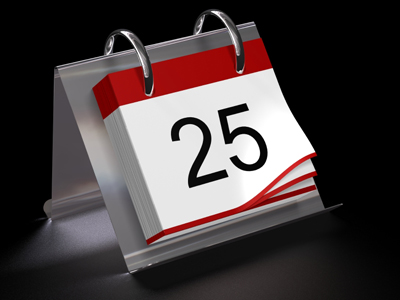To divide a number by 25: divide by 100, then multiply by 4.

# Multiplication and Division (Medium)

Multiplication and division is multiplying and dividing numbers. In this 11-plus Maths quiz, you'll learn a few techniques that will help you multiply and divide numbers more easily. Learn as many of these techniques as you think may be useful to you. Knowing your times tables is always useful when it comes to multiplying and dividing.

These questions are longer than usual as they have an extra tip added to them. You might have your own way of doing things, but if you learn the tips, it could improve your maths and make you twice as fast! Being able to calculate quickly isn't just for show; it comes in handy when you are out & about and haven't got the time or a pencil & paper to hand.

Take your time and if you want extra practise, try out some of the tips with other numbers too.

1.
Using the tip, what is the correct answer to the given calculation?
TIP: To multiply a number by 25: multiply by 100, then divide by 4.
816 × 25 = ?
24,000
20,040
20,400
20,004
816 × 25 = (816 × 100) × 4 = 81,600 ÷ 4 = 20,400
2.
Using the tip, what is the correct answer to the given calculation?
TIP: To multiply a number by another number which is a multiple of 10 and whose only non zero digit is the leading digit 1: move the digits to the left by the same number of zeros that are in the multiple of 10. Note: whole numbers that do not have decimal parts are written without a d.p. e.g. 200.0 = 200: in such cases the d.p. is not normally needed.
34.56 × 100 = ?
345.6
3,456
34,560
0.3456
34.56 × 100 = 3,456
3.
Using the tip, what is the correct answer to the given calculation?
TIP: To multiply a number by 5: first divide by 2, then move the digits to the left. Note: whole numbers that do not have decimal parts are written without a d.p. e.g. 200.0 = 200: in such cases the d.p. is not normally needed.
3,434 × 5 = ?
17,107
17,017
17,710
17,170
3,434 × 5 = (3,434 ÷ 2) = 1,717. Move the d.p. 1 place to the right gives 17,170. TIP: All odd numbers multiplied by 5 end in 5, and all even numbers multiplied by 5 end in 0
4.
Using the tip, what is the correct answer to the given calculation?
TIP: To multiply a number by 9: first multiply the number by 10, then subtract the original number from it.
47 × 9 = ?
432
423
342
324
47 × 9 = (47 × 10) - 47 = 423
5.
Using the tip, what is the correct answer to the given calculation?
TIP: To divide a number by 25: divide by 100, then multiply by 4.
225 ÷ 25 = ?
8
10
9
7
225 ÷ 25 = (225 ÷ 100) × 4 = 2.25 × 4 = 9
6.
Using the tip, what is the correct answer to the given calculation?
TIP: To divide a number by 5: first multiply by 2 and then move the digits to the right.
888 ÷ 5 = ?
17,760
17.76
1,776
177.6
888 ÷ 5 = (888 × 2) = 1,776. Move the d.p. 1 place to the left gives 177.6
7.
Using the tip, what is the correct answer to the given calculation?
TIP: To multiply a number by 8: multiply the number by 2, then by 2 again, and finally by 2.
120 × 8 = ?
609
906
960
690
120 × 2 = 240; 240 × 2 = 480; 480 × 2 = 960
8.
Using the tip, what is the correct answer to the given calculation?
TIP: To multiply a number by 11, first multiply the number by 10, then add the original number to it.
96 × 11 = ?
1,605
1,056
1,560
1,065
96 × 11 = (96 × 10) + 96 = 1,056
9.
Using the tip, what is the correct answer to the given calculation?
TIP: To divide a number by another number which is a multiple of 10 and whose only non zero digit is the leading digit 1: move the digits to the right by the same number of zeros that are in the multiple of 10. Note: whole numbers that do not have decimal parts are written without a d.p. e.g. 200.0 = 200: in such cases the d.p. is not normally needed.
76,345 ÷ 1,000 = ?
76.345
763.45
7,634.5
0.76345
76,345 = 76,345.0 so 76,345.0 ÷ 1,000 = 76.345: you have to put in the zero to show that there is no whole number part
10.
Using the tip, what is the correct answer to the given calculation?
TIP: To multiply a number by 12, first multiply the number by 10, then add twice the original number to it.
38 × 12 = ?
456
465
645
546
38 × 12 = (38 × 10) + (2 × 38) = 380 + 76 = 456
Author:  Frank Evans